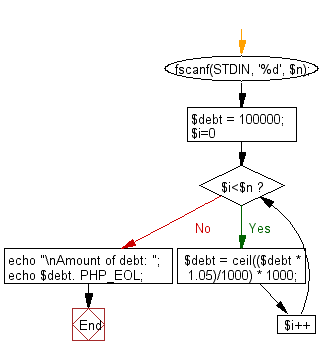﻿ PHP Exercise: Compute the amount of the debt in n months - w3resource

# PHP Exercises: Compute the amount of the debt in n months

## PHP: Exercise-50 with Solution

Write a PHP program to compute the amount of the debt in n months. The borrowing amount is \$100,000 and the loan adds 5% interest of the debt and rounds it to the nearest 1,000 above month by month.

Input:
An integer n (0 ≤ n ≤ 100) .

Sample Solution: -

PHP Code:

``````<?php
fscanf(STDIN, '%d', \$n);
\$debt = 100000;

for(\$i=0; \$i<\$n; \$i++){
\$debt = ceil((\$debt * 1.05)/1000) * 1000;
}
echo "\nAmount of debt: ";
echo \$debt. PHP_EOL;
?>
```
```

Sample Output:

```Amount of debt: 137000
```

Flowchart:PHP Code Editor:

Have another way to solve this solution? Contribute your code (and comments) through Disqus.

What is the difficulty level of this exercise?

Test your Programming skills with w3resource's quiz.

﻿

## PHP: Tips of the Day

Returns all elements in an array except for the first one

Example:

```<?php
function tips_tail(\$items)
{
return count(\$items) > 1 ? array_slice(\$items, 1) : \$items;
}

print_r(tips_tail([1, 5, 7]));
?>
```

Output:

```Array
(
 => 5
 => 7
)
```